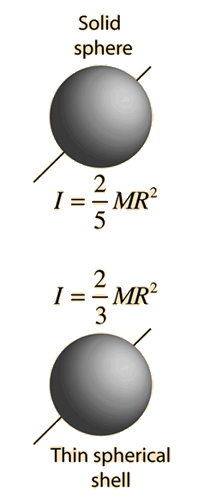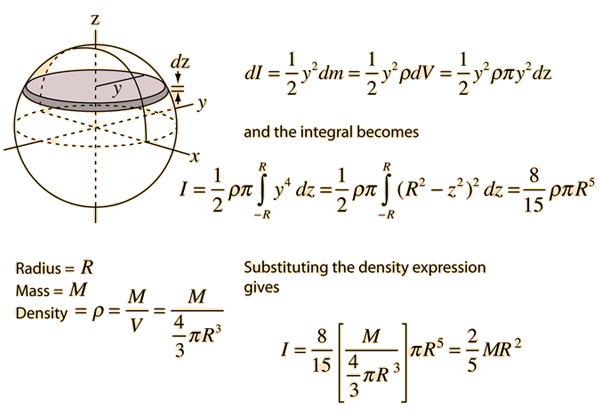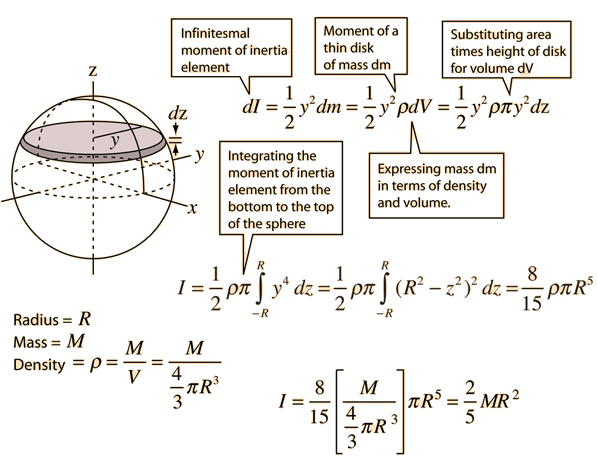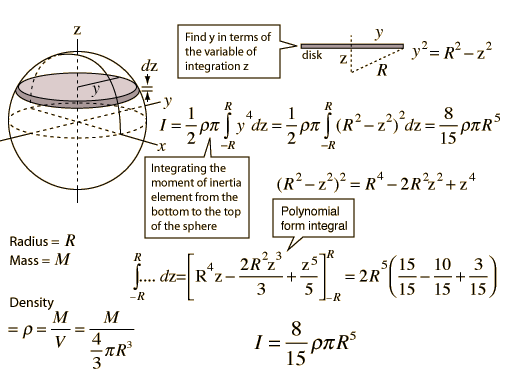# Moment of Inertia: SphereThe moment of inertia of a sphere about its central axis and a thin spherical shell are shown.

For mass M = kg
the moment of inertia of a solid sphere is
I(solid sphere) = kg m2
and the moment of inertia of a thin spherical shell is
I(spherical shell) = kg m2

### Show development of expressions

Index

Moment of inertia concepts

 HyperPhysics***** Mechanics R Nave
Go Back

# Moment of Inertia: Sphere

The expression for the moment of inertia of a sphere can be developed by summing the moments of infintesmally thin disks about the z axis. The moment of inertia of a thin disk isIndex

Moment of inertia concepts
Go Back

# Sphere Details

Details about the moment of inertia of a sphere.Index

Moment of inertia concepts

 HyperPhysics***** Mechanics R Nave
Go Back

# Sphere Integral Details

Details about the moment of inertia of a sphere.The process involves integrating the moments of inertia of infinitesmally thin disks from the top to the bottom of the sphere. This involves polynomial form integrals.

Index

Moment of inertia concepts

 HyperPhysics***** Mechanics R Nave
Go Back